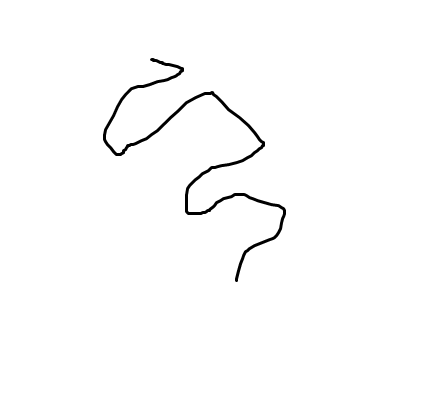# Mouse Dragging

If I want to click on the LMB and while I am holding it, move the mouse in any direction, I need to be able to tell which direction and how many units the Mouse was dragged before release. How to do it?

Thanks …

Do you want to get the direction and distance from the mouse since you pressed it? I get you?To check it while the mouse button is pressed:

To check it once (when the mouse button is released):

Since, to calculate the distance between i[/i] and i[/i]: ``` Distance = sqrt( (a-x)^2 + (b-y)^2 ) ```
and to calculate the angle between i[/i] and i[/i]: ``` Angle = atan2( (b-y) , (a-x) ) ```

Incredible and complex at same time kkk’

Motivated me to study mathematics to build complex codes… hard task

Ok, I think I got simpler solution, but it will only work in 8 directions (you would only be able to tell if mouse moved up, down, left, right or in diagonals).

If you’re okay with this, here it is.

First you’ll need 4 variables.

• When mouse is pressed down, you save initial mouse position.
• When mouse is being let go, you save final position.
Now you need to compare.
• When original x is smaller than final, mouse moved to the right
• When original x is bigger than final, mouse moved to the left
• When original y is smaller than final, mouse moved to the down
• When original y is bigger than final, mouse moved to the up
• When original x is smaller than final and original y is smaller than final and difference on both axes is bigger than certain value (to avoid erroneously interpreting straight moves like diagonal, you need to come up with proper value for this deadzone) it was moved to the down-right.
• When original x is bigger than final and original y is bigger than final and difference on both axes is bigger than certain value (to avoid erroneously interpreting straight moves like diagonal, you need to come up with proper value for this deadzone) it was moved to the up-left.
• When original x is bigger than final and original y is smaller than final and difference on both axes is bigger than certain value (to avoid erroneously interpreting straight moves like diagonal, you need to come up with proper value for this deadzone) it was moved to the down-left.
• When original x is smaller than final and original y is bigger than final and difference on both axes is bigger than certain value (to avoid erroneously interpreting straight moves like diagonal, you need to come up with proper value for this deadzone) it was moved to the up-right.

Kinda wall of text, but concept is really simple once you read it and try.

If you want just the absolute values (how far is it from the point you started to the point you finished at), there is yet another alternate method.

``` [u]Conditions: [/u] LMB is pressed Variable x = 0 [u]Actions: [/u] Create an object lastmousepos at Mouse(X,""), Mouse(Y,"") Do = 1 to Variable x ```

``` [u]Conditions: [/u] LMB is NOT pressed Variable x = 1 [u]Actions: [/u] Create an object endmousepos at Mouse(X,""), Mouse(Y,"") Do = 1 to Variable x ```

And then you can compare the distance and angle between objects lastmousepos and endmousepos.

If you want to know very accurately how may units were traveled in total, then you could create a waypoint every x seconds (or even frame) and then calculate the distance from the last waypoint while the mouse is pressed. This is important if you want to know how far I traveled and I took a route like the one in the attached picture.Thank you everyone, the solution is definitely complex, I will try them out and see which goes the best …